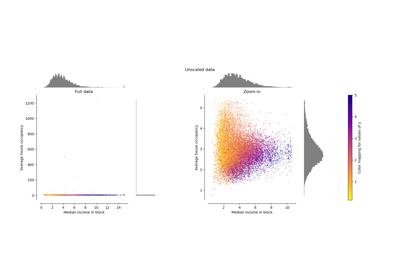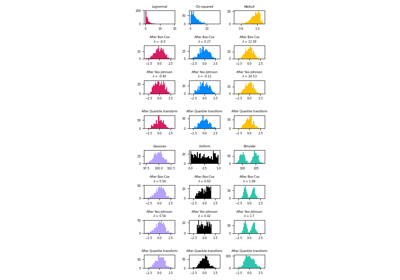# sklearn.preprocessing.PowerTransformer¶

class sklearn.preprocessing.PowerTransformer(method='yeo-johnson', *, standardize=True, copy=True)[source]

Apply a power transform featurewise to make data more Gaussian-like.

Power transforms are a family of parametric, monotonic transformations that are applied to make data more Gaussian-like. This is useful for modeling issues related to heteroscedasticity (non-constant variance), or other situations where normality is desired.

Currently, PowerTransformer supports the Box-Cox transform and the Yeo-Johnson transform. The optimal parameter for stabilizing variance and minimizing skewness is estimated through maximum likelihood.

Box-Cox requires input data to be strictly positive, while Yeo-Johnson supports both positive or negative data.

By default, zero-mean, unit-variance normalization is applied to the transformed data.

For an example visualization, refer to Compare PowerTransformer with other scalers. To see the effect of Box-Cox and Yeo-Johnson transformations on different distributions, see: Map data to a normal distribution.

Read more in the User Guide.

New in version 0.20.

Parameters:
method{‘yeo-johnson’, ‘box-cox’}, default=’yeo-johnson’

The power transform method. Available methods are:

• ‘yeo-johnson’ , works with positive and negative values

• ‘box-cox’ , only works with strictly positive values

standardizebool, default=True

Set to True to apply zero-mean, unit-variance normalization to the transformed output.

copybool, default=True

Set to False to perform inplace computation during transformation.

Attributes:
lambdas_ndarray of float of shape (n_features,)

The parameters of the power transformation for the selected features.

n_features_in_int

Number of features seen during fit.

New in version 0.24.

feature_names_in_ndarray of shape (n_features_in_,)

Names of features seen during fit. Defined only when X has feature names that are all strings.

New in version 1.0.

power_transform

Equivalent function without the estimator API.

QuantileTransformer

Maps data to a standard normal distribution with the parameter output_distribution='normal'.

Notes

NaNs are treated as missing values: disregarded in fit, and maintained in transform.

References

Examples

>>> import numpy as np
>>> from sklearn.preprocessing import PowerTransformer
>>> pt = PowerTransformer()
>>> data = [[1, 2], [3, 2], [4, 5]]
>>> print(pt.fit(data))
PowerTransformer()
>>> print(pt.lambdas_)
[ 1.386... -3.100...]
>>> print(pt.transform(data))
[[-1.316... -0.707...]
[ 0.209... -0.707...]
[ 1.106...  1.414...]]


Methods

 fit(X[, y]) Estimate the optimal parameter lambda for each feature. fit_transform(X[, y]) Fit PowerTransformer to X, then transform X. get_feature_names_out([input_features]) Get output feature names for transformation. Get metadata routing of this object. get_params([deep]) Get parameters for this estimator. Apply the inverse power transformation using the fitted lambdas. set_output(*[, transform]) Set output container. set_params(**params) Set the parameters of this estimator. Apply the power transform to each feature using the fitted lambdas.
fit(X, y=None)[source]

Estimate the optimal parameter lambda for each feature.

The optimal lambda parameter for minimizing skewness is estimated on each feature independently using maximum likelihood.

Parameters:
Xarray-like of shape (n_samples, n_features)

The data used to estimate the optimal transformation parameters.

yNone

Ignored.

Returns:
selfobject

Fitted transformer.

fit_transform(X, y=None)[source]

Fit PowerTransformer to X, then transform X.

Parameters:
Xarray-like of shape (n_samples, n_features)

The data used to estimate the optimal transformation parameters and to be transformed using a power transformation.

yIgnored

Not used, present for API consistency by convention.

Returns:
X_newndarray of shape (n_samples, n_features)

Transformed data.

get_feature_names_out(input_features=None)[source]

Get output feature names for transformation.

Parameters:
input_featuresarray-like of str or None, default=None

Input features.

• If input_features is None, then feature_names_in_ is used as feature names in. If feature_names_in_ is not defined, then the following input feature names are generated: ["x0", "x1", ..., "x(n_features_in_ - 1)"].

• If input_features is an array-like, then input_features must match feature_names_in_ if feature_names_in_ is defined.

Returns:
feature_names_outndarray of str objects

Same as input features.

Get metadata routing of this object.

Please check User Guide on how the routing mechanism works.

Returns:

A MetadataRequest encapsulating routing information.

get_params(deep=True)[source]

Get parameters for this estimator.

Parameters:
deepbool, default=True

If True, will return the parameters for this estimator and contained subobjects that are estimators.

Returns:
paramsdict

Parameter names mapped to their values.

inverse_transform(X)[source]

Apply the inverse power transformation using the fitted lambdas.

The inverse of the Box-Cox transformation is given by:

if lambda_ == 0:
X = exp(X_trans)
else:
X = (X_trans * lambda_ + 1) ** (1 / lambda_)


The inverse of the Yeo-Johnson transformation is given by:

if X >= 0 and lambda_ == 0:
X = exp(X_trans) - 1
elif X >= 0 and lambda_ != 0:
X = (X_trans * lambda_ + 1) ** (1 / lambda_) - 1
elif X < 0 and lambda_ != 2:
X = 1 - (-(2 - lambda_) * X_trans + 1) ** (1 / (2 - lambda_))
elif X < 0 and lambda_ == 2:
X = 1 - exp(-X_trans)

Parameters:
Xarray-like of shape (n_samples, n_features)

The transformed data.

Returns:
Xndarray of shape (n_samples, n_features)

The original data.

set_output(*, transform=None)[source]

Set output container.

See Introducing the set_output API for an example on how to use the API.

Parameters:
transform{“default”, “pandas”}, default=None

Configure output of transform and fit_transform.

• "default": Default output format of a transformer

• "pandas": DataFrame output

• None: Transform configuration is unchanged

Returns:
selfestimator instance

Estimator instance.

set_params(**params)[source]

Set the parameters of this estimator.

The method works on simple estimators as well as on nested objects (such as Pipeline). The latter have parameters of the form <component>__<parameter> so that it’s possible to update each component of a nested object.

Parameters:
**paramsdict

Estimator parameters.

Returns:
selfestimator instance

Estimator instance.

transform(X)[source]

Apply the power transform to each feature using the fitted lambdas.

Parameters:
Xarray-like of shape (n_samples, n_features)

The data to be transformed using a power transformation.

Returns:
X_transndarray of shape (n_samples, n_features)

The transformed data.

## Examples using sklearn.preprocessing.PowerTransformer¶Compare the effect of different scalers on data with outliers

Compare the effect of different scalers on data with outliersMap data to a normal distribution

Map data to a normal distribution